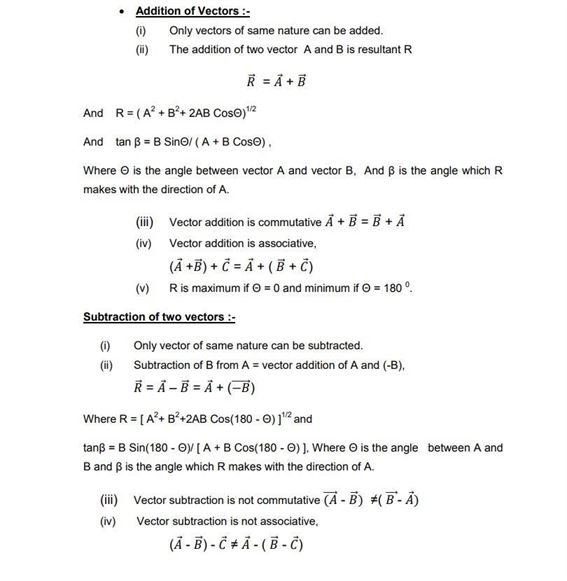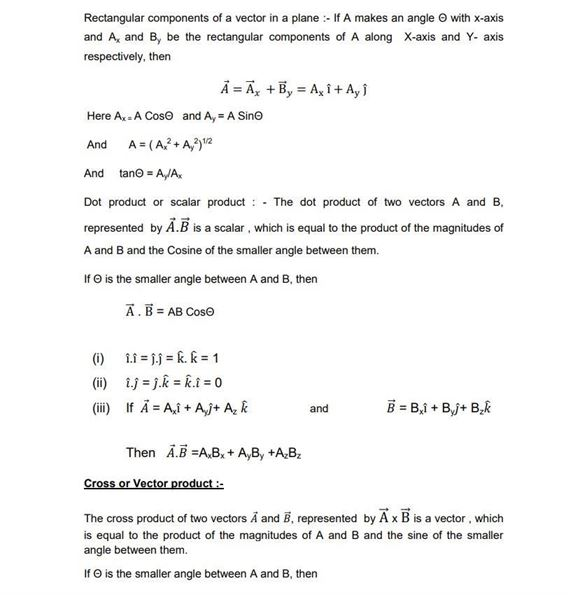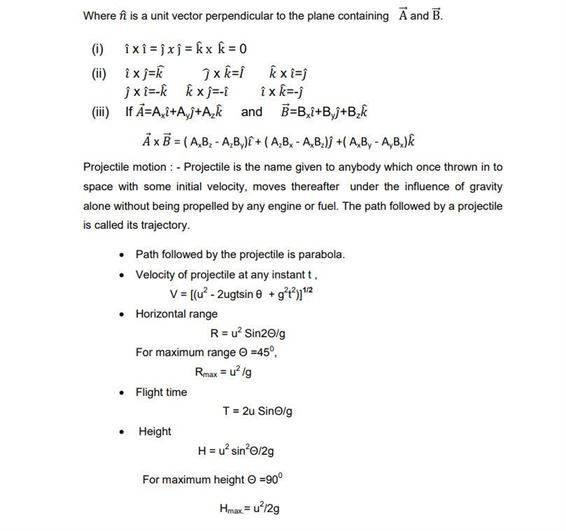# Physics Revision Notes For Class 11 Chapter 4 Motion in a Plane

Students can find the class 11 physics revision notes chapter 4 motion in a plane here. These notes comprise all the explanation with short notes about motion. They will find the difference between scalar and vector quantities and along with that, they will find the definition of various terms such as unit, coplanar and displacement vectors etc. This note provides important formulas such as triangle law of vector addition, polygon law of vector addition.and also see some theorems such as lami’s theorem along with definition on angular acceleration, angular velocity, centripetal acceleration etc. These notes are very important for preparation in exams.

See the Class 11 Revision notes for physics chapter 4 motion in a plane below.